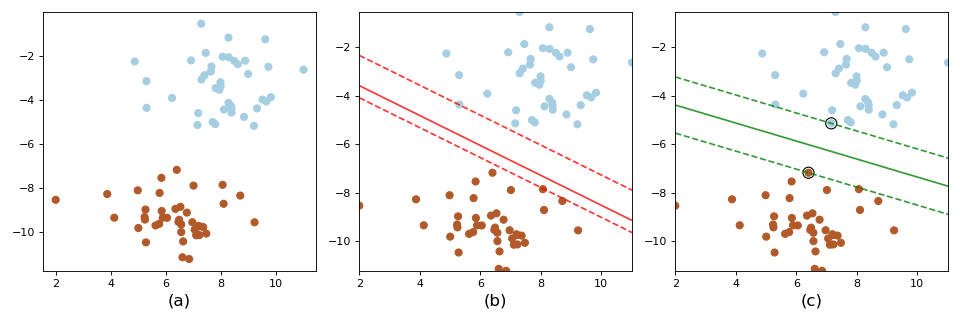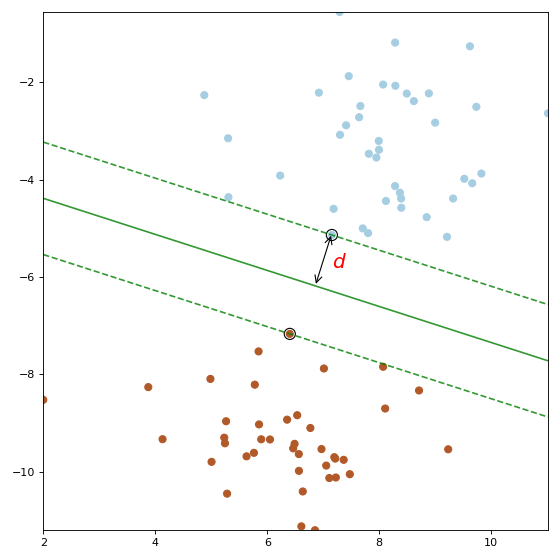# 从另一个角度看支持向量机

## 1 什么是支持向量机SVM算法认为分类器c在性能上要优于分类器b，其依据是c的分类间隔比b要大。所谓分类间隔是指：在保证决策面方向不变且不会出现错分样本的情况下移动决策面，然后在原来决策面的两侧会找到两个极限位置（越过该位置就会产生错分现象），而决策面到极限位置之间的距离就是分类间隔，即决策面到两条虚线之间的垂直距离就是这个策面对应的分类间隔。但显然每一个可能把数据集正确分开的决策面所对应的分类间隔通常都是不同的，而那个具有“最大间隔”的决策面就是SVM要寻找的最优解。那什么又叫“最大间隔”呢？

## 2 SVM的数学建模

①目标函数，也就是你希望什么东西的什么指标达到最好；

②优化对象，你期望通过改变哪些因素来使你的目标函数达到最优。

### 2.1 决策面

y = k x + b (1) y=kx+b\tag {1}

k x 1 + ( − 1 ) x 2 + b = 0 (2) kx_1+(-1)x_2+b=0\tag{2}

[ k , − 1 ] [ x 1 x 2 ] + b = 0 (3) [k,-1]\left[ \begin{array}{c}x_1\\x_2\end{array} \right] +b=0 \tag{3}

w T x + b = 0 (4) w^Tx+b=0\tag{4}

### 2.2 分类间隔d = ∣ w T x + b ∣ ∣ ∣ w ∣ ∣ (5) d = \frac{|w^Tx+b|}{||w||} \tag{5}

### 2.3 约束条件

①如何保证一条直线能够将所有的样本点都正确分类？

②即便找到了正确的决策面方向，还要保证决策面的位置应该在间隔区域的中轴线上，所以用来确定决策面位置的截距 b b 也应该受到决策面方向和样本点分布的约束。

③即便取到了合适的方向和截距，公式 ( 5 ) (5) 里面的 x x 不应该是随随便便的一个样本点，而是支持向量对应的样本点。对于一个给定的决策面，我们该如何找到对应的支持向量？

y ( i ) = { + 1 for blue points − 1 for brown points (6) y^{(i)} = \left\{\begin{array}{ll}+1 & \text{for blue points}\\-1 & \text{for brown points}\end{array}\right.\tag{6}

{ w T x ( i ) + b > 0 ; y ( i ) = + 1 w T x ( i ) + b < 0 ; y ( i ) = − 1 (7) \left\{\begin{array}{ll} w^Tx^{(i)}+b>0; & y^{(i)}=+1\\ w^Tx^{(i)}+b<0; &y^{(i)}=-1\end{array}\right.\tag{7}

{ w T x ( i ) + b ∣ ∣ w ∣ ∣ ≥ d ; y ( i ) = + 1 w T x ( i ) + b ∣ ∣ w ∣ ∣ ≤ − d ; y ( i ) = − 1 (8) \left\{\begin{array}{ll} \frac{w^Tx^{(i)}+b}{||w||}\geq d; & y^{(i)}=+1\\[2ex] \frac{w^Tx^{(i)}+b}{||w||}\leq -d; &y^{(i)}=-1\end{array}\right.\tag{8}

{ w d T x ( i ) + b d ≥ 1 ; y ( i ) = 1 w d T x ( i ) + b d ≤ − 1 ; y ( i ) = − 1 (9) \left\{\begin{array}{ll} w_d^Tx^{(i)}+b_d\geq 1; & y^{(i)}=1\\ w_d^Tx^{(i)}+b_d\leq -1; & y^{(i)}=-1\end{array}\right.\tag{9}

w d = w ∣ ∣ w ∣ ∣ d ,      b d = b ∣ ∣ w ∣ ∣ d (10) w_d = \frac{w}{||w||d},\;\; b_d = \frac{b}{||w||d}\tag{10}

{ w T w ( i ) + b ≥ 1 ; y ( i ) = 1 w T x ( i ) + b ≤ − 1 ; y ( i ) = − 1 (11) \left\{\begin{array}{ll} w^Tw^{(i)}+b\geq 1; & y^{(i)}=1\\ w^Tx^{(i)}+b\leq -1; & y^{(i)}=-1\end{array}\right.\tag{11}

### 2.4 最大化分类间隔

d = ∣ w T x ( i ) + b ∣ ∣ ∣ w ∣ ∣ = 1 ∣ ∣ w ∣ ∣ ,      if    x ( i )    is a support vector (12) d = \frac{|w^Tx^{(i)}+b|}{||w||}=\frac{1}{||w||},\;\;\textrm{if} \;x^{(i)}\; \textrm {is a support vector} \tag{12}

y ( i ) ( w T x ( i ) + b ) ≥ 1 ,    i = 1 , 2 , . . . m (13) y^{(i)}(w^Tx^{(i)}+b)\geq 1,\;i=1,2,...m\tag{13}

min ⁡ w , b 1 2 ∣ ∣ w ∣ ∣ 2 s . t .      y ( i ) ( w T x ( i ) + b ) ≥ 1 , i = 1 , 2 , . . . m (14) \begin{aligned} &\min_{w,b} \frac{1}{2}{||w||^2}\\[1ex] &s.t.\;\;y^{(i)}\large(w^Tx^{(i)}+b)\geq1,i=1,2,...m \end{aligned}\tag{14}

## 3 总结

### 引用

 零基础学SVM https://zhuanlan.zhihu.com/p/24638007

 示例代码：关注公众号回复“示例代码”即可直接获取！05-292万+
11-2464306-241万+
03-292万+
05-283万+
02-195878
11-173万+
06-303万+
05-159186
02-254万+
03-191601
01-021万+
07-111万+
09-091万+
01-155882
03-315万+
11-202万+
12-076768
03-191万+
12-273万+